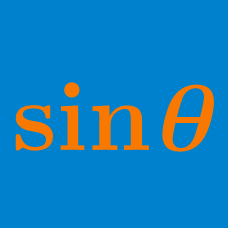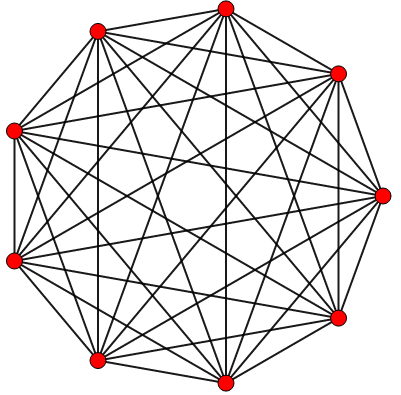Geometry

# Trigonometric Functions: Level 4 Challenges

$\displaystyle \sum_{k=1}^{145} \left [ \sin \left ( \frac {2\pi k}{8} \right ) - i \ \cos \left ( \frac {2\pi k}{14} \right ) \right ] = \frac {1}{\sqrt{a}} - i \cos \left ( \frac {\pi}{b} \right ).$ Find the sum of the positive integers $a$ and $b.$

Note: $i = \sqrt{-1}.$$\large \sec^{2} \left ( \dfrac{\pi}{9} \right ) + \sec^{2} \left (\dfrac{2 \pi}{9}\right) + \sec^{2} \left (\dfrac{4 \pi}{9}\right) = \, ?$

Evaluate $\large \sum_{m=1}^{\infty} \tan^{-1} \left(\frac{8m}{16m^4-32m^2+5}\right).$

Remember that the range of the inverse tangent function is $( - \frac{ \pi}{2} , \frac{ \pi }{2})$.

If the value of $\sin^{24}\frac{\pi}{24} + \cos^{24}\frac{\pi}{24}$

is expressed in simplest form as $\dfrac{a + b\sqrt{c}}{d},$ find the last three digits of $a + b + c + d.$

Note: Simplest form refers to the condition that $a,b,c,d$ are positive integers with $\gcd(a,b,d)=1$ and $c$ is not divisible by the square of any prime.

If $\displaystyle\sum_{k=1}^{89} \cos^{6}(k^{\circ}) = \frac{a}{b}$, where $a$ and $b$ are positive coprime integers, then find $a + b.$

×

Problem Loading...

Note Loading...

Set Loading...